Back to Results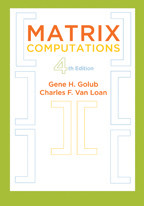# Matrix Computations

Gene H. Golub and Charles F. Van Loan

fourth edition
Publication Date
Request Exam CopyRequest Review Copy

A comprehensive treatment of numerical linear algebra from the standpoint of both theory and practice.

The fourth edition of Gene H. Golub and Charles F. Van Loan's classic is an essential reference for computational scientists and engineers in addition to researchers in the numerical linear algebra community. Anyone whose work requires the solution to a matrix problem and an appreciation of its mathematical properties will find this book to be an indispensible tool.

This revision is a cover-to-cover expansion and renovation of the third edition. It now includes an introduction to tensor…

A comprehensive treatment of numerical linear algebra from the standpoint of both theory and practice.

The fourth edition of Gene H. Golub and Charles F. Van Loan's classic is an essential reference for computational scientists and engineers in addition to researchers in the numerical linear algebra community. Anyone whose work requires the solution to a matrix problem and an appreciation of its mathematical properties will find this book to be an indispensible tool.

This revision is a cover-to-cover expansion and renovation of the third edition. It now includes an introduction to tensor computations and brand new sections on
• fast transforms
• parallel LU
• discrete Poisson solvers
• pseudospectra
• structured linear equation problems
• structured eigenvalue problems
• large-scale SVD methods
• polynomial eigenvalue problems

Matrix Computations is packed with challenging problems, insightful derivations, and pointers to the literature—everything needed to become a matrix-savvy developer of numerical methods and software. The second most cited math book of 2012 according to MathSciNet, the book has placed in the top 10 for since 2005.

Matrix Computations

Gene H. Golub and Charles F. Van Loan

fourth edition
Publication Date
Request Exam CopyRequest Review Copy
Related

## Related Books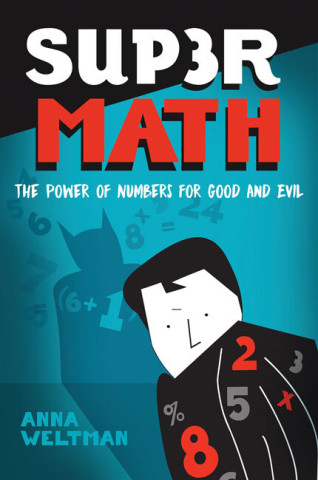Supermath

Anna Weltman

\$24.95
Supermath

Anna Weltman

Publication Date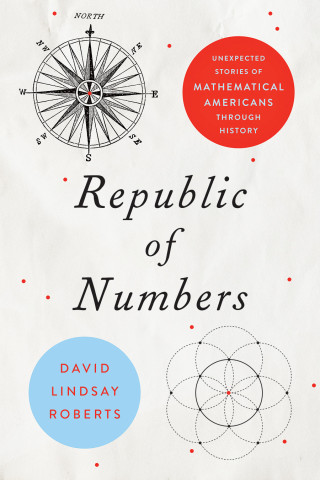Republic of Numbers

David Lindsay Roberts

\$29.95
Republic of Numbers

David Lindsay Roberts

Publication Date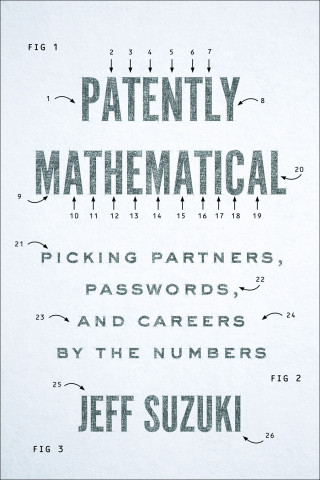Patently Mathematical

Jeff Suzuki

\$34.95
Patently Mathematical

Jeff Suzuki

Publication Date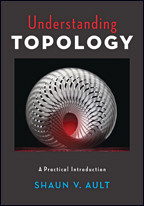Understanding Topology

Shaun V. Ault

\$99.95
Understanding Topology

Shaun V. Ault

Publication DateCalculus in Context

Alexander J. Hahn

\$95.00
Calculus in Context

Alexander J. Hahn

Publication Date
Reviews

## Reviews

Problems, solutions, and discussions of the formulas, methods and literature surrounding matrix computations make for a reference that is specific and well detailed: perfect for any college-level math collection appealing to engineers.

Written for scientists and engineers, Matrix Computations provides comprehensive coverage of numerical linear algebra. Anyone whose work requires the solution to a matrix problem and an appreciation of mathematical properties will find this book to be an indispensable tool.

A mine of insight and information and a provocation to thought; the annotated bibliographies are helpful to those wishing to explore further. One could not ask for more, and the book should be considered a resounding success.

## Book Details

Publication Date
Status
Available
Trim Size
7
x
10
Pages
784
ISBN
9781421407944
Illustration Description
31 line drawings
Subject

Preface
Global References
Other Books
Useful URLs
Common Notation
Chapter 1. Matrix Multiplication
1.1. Basic Algorithms and Notation
1.2. Structure and Efficiency
1.3. Block Matrices and Algorithms
1.4. Fast

Preface
Global References
Other Books
Useful URLs
Common Notation
Chapter 1. Matrix Multiplication
1.1. Basic Algorithms and Notation
1.2. Structure and Efficiency
1.3. Block Matrices and Algorithms
1.4. Fast Matrix-Vector Products
1.5. Vectorization and Locality
1.6. Parallel Matrix Multiplication
Chapter 2. Matrix Analysis
2.1. Basic Ideas from Linear Algebra
2.2. Vector Norms
2.3. Matrix Norms
2.4. The Singular Value Decomposition
2.5. Subspace Metrics
2.6. The Sensitivity of Square Systems
2.7. Finite Precision Matrix Computations
Chapter 3. General Linear Systems
3.1. Triangular Systems
3.2. The LU Factorization
3.3. Roundoff Error in Gaussian Elimination
3.4. Pivoting
3.5. Improving and Estimating Accuracy
3.6. Parallel LU
Chapter 4. Special Linear Systems
4.1. Diagonal Dominance and Symmetry
4.2. Positive Definite Systems
4.3. Banded Systems
4.4. Symmetric Indefinite Systems
4.5. Block Tridiagonal Systems
4.6. Vandermonde Systems
4.7. Classical Methods for Toeplitz Systems
4.8. Circulant and Discrete Poisson Systems
Chapter 5. Orthogonalization and Least Squares
5.1. Householder and Givens Transformations
5.2. The QR Factorization
5.3. The Full-Rank Least Squares Problem
5.4. Other Orthogonal Factorizations
5.5. The Rank-Deficient Least Squares Problem
5.6. Square and Underdetermined Systems
Chapter 6. Modified Least Squares Problems and Methods
6.1. Weighting and Regularization
6.2. Constrained Least Squares
6.3. Total Least Squares
6.4. Subspace Computations with the SVD
6.5. Updating Matrix Factorizations
Chapter 7. Unsymmetric Eigenvalue Problems
7.1. Properties and Decompositions
7.2. Perturbation Theory
7.3. Power Iterations
7.4. The Hessenberg and Real Schur Forms
7.5. The Practical QR Algorithm
7.6. Invariant Subspace Computations
7.7. The Generalized Eigenvalue Problem
7.8. Hamiltonian and Product Eigenvalue Problems
7.9. Pseudospectra
Chapter 8. Symmetric Eigenvalue Problems
8.1. Properties and Decompositions
8.2. Power Iterations
8.3. The Symmetric QR Algorithm
8.4. More Methods for Tridiagonal Problems
8.5. Jacobi Methods
8.6. Computing the SVD
8.7. Generalized Eigenvalue Problems with Symmetry
Chapter 9. Functions of Matrices
9.1. Eigenvalue Methods
9.2. Approximation Methods
9.3. The Matrix Exponential
9.4. The Sign, Square Root, and Log of a Matrix
Chapter 10. Large Sparse Eigenvalue Problems
10.1. The Symmetric Lanczos Process
10.3. Practical Lanczos Procedures
10.4. Large Sparse SVD Frameworks
10.5. Krylov Methods for Unsymmetric Problems
10.6. Jacobi-Davidson and Related Methods
Chapter 11. Large Sparse Linear System Problems
11.1. Direct Methods
11.2. The Classical Iterations
11.4. Other Krylov Methods
11.5. Preconditioning
11.6. The Multigrid Framework
Chapter 12. Special Topics
12.1. Linear Systems with Displacement Structure
12.2. Structured-Rank Problems
12.3. Kronecker Product Computations
12.4. Tensor Unfoldings and Contractions
12.5. Tensor Decompositions and Iterations
Index

Author Bios
Featured Contributor

## Gene H. Golub

Gene H. Golub (1932–2007) was a professor emeritus and former director of scientific computing and computational mathematics at Stanford University.
Featured Contributor

Resources